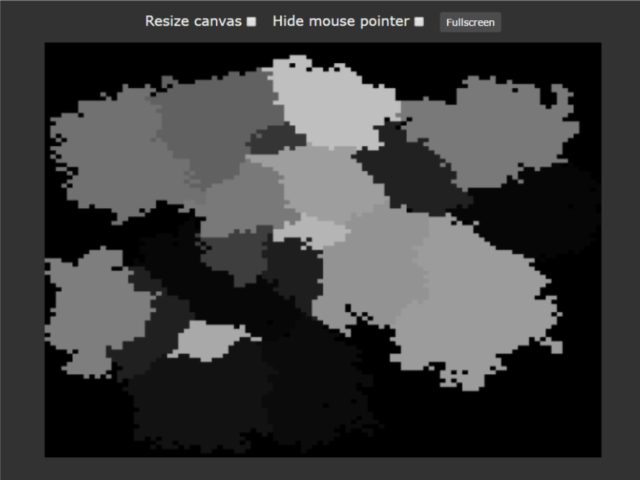-=+=- -=+=- -=+=- -=+=- -=+=- -=+=- -=+=- -=+=- -=+=- -=+=- -=+=- -=+=- -=+=- -=+=- -=+=- -=+=- -=+=- -=+=- -=+=- -=+=- -=+=- -=+=- -=+=- -=+=- -=+=- -=+=- -=+=- -=+=- -=+=- -=+=- (c) WidthPadding Industries 1987 0|582|0 -=+=- -=+=- -=+=- -=+=- -=+=- -=+=- -=+=- -=+=- -=+=- -=+=- -=+=- -=+=- -=+=- -=+=- -=+=- -=+=- -=+=- -=+=- -=+=- -=+=- -=+=- -=+=- -=+=- -=+=- -=+=- -=+=- -=+=- -=+=- -=+=- -=+=-
SoCoder -> Snippet Home -> Generators

PakzCreated : 07 December 2016
Edited : 07 December 2016
Language : Other

### Bush fire maps

Create countries or provincesHere the emscripten page :
https://cromdesi.home.xs4all.nl/emscripten/bushfire/Untitled1.html

Here a youtube video of it :
https://youtu.be/VOVi-KdhQ5c

I found this method online years ago. What it does is find a position on the map and see if it can burn a position next near it. So creating a surface. Here I made it so it makes different style maps each time. I made it so it goes slowly from empty to a filled screen.

Would also be changeable to screen dissolve.

Press mouse (tap) on the map to create new map.

Code is monkey 2.